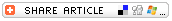## Search formIntroducing Calculators:
A K-2 Computer ActivitySubjects

• Mathematics
--Arithmetic

• K-2

Brief Description

Students work in small groups to record how many pets each has, then use calculators to find the total number of pets owned by students in the entire group.

Objectives

Students will

• practice basic addition skills on a worksheet and with a calculator.
• practice calculator skills.
• practice computer skills.

Keywords

calculators, mathematics

• Student access to a calculators on a computer (or an actual calculator).
• Projector or TV monitor for demonstration.

Lesson Plan

Preparation

• Print a copy of the downloadable worksheet for each student. The lesson assumes basic addition skills (adding to 20). Set up projector or TV monitor.
• Open the calculator on student computers (if using a computer calculator). On a PC, you'll find the calculator by clicking Start>All Programs (or Programs)>Accessories>Calculator. On a Mac, open the hard drive icon, click Applications>Calculator. If using Macs running OS 10, when you find the calculator icon in the Applications folder, just drag it to the Dock -- or move it to the Launcher in earlier OS versions

Introduction

• Introduce the lesson by displaying a calculator and asking students what it is. After the class identifies it and discusses what it is used for, show students a calculator on a computer. Explain that both calculators do the same thing -- quickly add, subtract, and perform other calculations with numbers, especially very large numbers.
• Explain to students that they will use a calculator to find out how many pets and what kinds of pets they own.

Process

• Arrange students into groups of 4.
• Distribute to each student a copy of the "How Many Pets?" worksheet. The worksheet requires students to write down the number of pets each member of the group owns, and shade in the calculator keys they would press to find the total number of pets owned by students in the entire group. Students then use a working calculator to find the sum.
• Walk students through the activity, perhaps using one group as an example. Be sure to show students how to shade in the calculator's buttons with their pencils, and remind them to press the plus and equals signs on the actual calculators to find the sum.
• Students should complete at least the first steps of the worksheet in a single sitting. You can opt to complete steps 4-6 at another time.
• Have students should turn in their worksheets at the end of the activity.

Extension

• Have students use their calculators to find the total number of pets owned by the entire class.
• Use the data collected to create basic graphs in Excel. Have students color in printed spreadsheets or watch as you create a basic graph. See Create Graphs and Charts with Excel if you are a beginning Excel user.

Assessment

Students will be evaluated on

• successful completion of the worksheet.
• observed work with the calculator.
• observed small group interaction.

Lesson Plan Source

Education World

Submitted By

Lorrie Jackson

National Standards

MATHEMATICS: Number and Operations